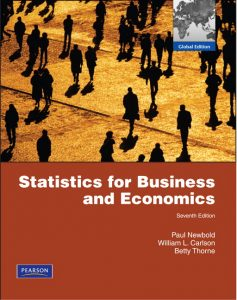28
Jan 17

## Review of Newbold, Carlson and Thorne###Review of Newbold, Carlson and Thorne "Statistics for Business and Economics", Pearson, 7th edition, 2010

Paul Newbold, the senior author, was a British economist known for his contributions to econometrics and time series analysis. His most famous contribution was a 1974 paper co-authored with Clive Granger, Nobel laureate, which introduced the concept of cointegration.

In addition to usual AP Stats topics, the book contains chapters on nonparametric estimation, comparison of subpopulation means, forecasting with time series models and introduction to decision theory. Overall, the level is higher than AP Stats. This textbook is required for University of London Statistics courses.

### What I like

There is no pointless, distracting talk so often seen in AP Stats texts. Except for a couple of definitions in the first two (elementary) chapters, the exposition is clear.

The book attempts to introduce the reader to algebra gently, through verbal statements of formulas, but quickly (in Chapter 2) switches to a mathematical notation that I find more adequate for AP Stats.

The probabilities are given where they are supposed to, right after using graphs and numerical measures to describe the data.

There are two types of exercises. End-of-section exercises are accessible to AP Stats students. The majority of end-of-chapter ones are much more complex. For example, Exercise 5.96 requires an explicit application of properties of three distributions: Bernoulli, binomial and normal. I learned a lot while solving exercises with my students. On one occasion the theory given in the book was insufficient, my students found on the Internet the missing part and explained the solution to me (it was about Poisson processes).

Among the applications of regression, there is the beta measure of financial risk.

### What I don't like

On the theory side the book may be satisfactory for AP Stats but not for the University of London. There are rudimentary proofs here and there; however, in general it's the same recipe approach. After two years of teaching following this approach I became convinced that it doesn't work and wrote my book.Figure 10.5. Flow Chart for Selecting the Appropriate Hypothesis test When Comparing Two Population Means

In case of means, the breakage of logical links is seen especially well. Different means are given in different places, and the students don't see how they are related to one another.

The proof that the sample mean unbiasedly estimates the population mean is relatively advanced. Students should be gradually prepared for proofs this complex. Since there is no systematic algebraic training, most students do not understand the proof given in the Appendix to Chapter 6.

Figures 9.10 and 10.5 for choosing the appropriate decision rules are definitely helpful but who is going to remember them without derivations?

### Conclusion: thumbs up!

The "Business" part of the name of the book may be misleading. This is not a book for those who want to understand just the basics of Statistics and/or to pass AP Stats because it is a required course. For future economists, this is an excellent introduction to Statistics, with two caveats. Firstly, while the selection and variety of exercises is superb, the theoretical part does not provide an adequate foundation and is better replaced by my book. Secondly, there is too much material even for a one-year course.

This book can be used by an instructor who is not constrained by the College Board curriculum. Since in our program we have a strong Econometrics course, Chapters 12, 13, 16 should be skipped. On the other hand, almost all material from Chapters 1-11 should be given with derivations. When students have access to Excel or Internet, instead of statistical tables students can use Excel or Mathematica functions.